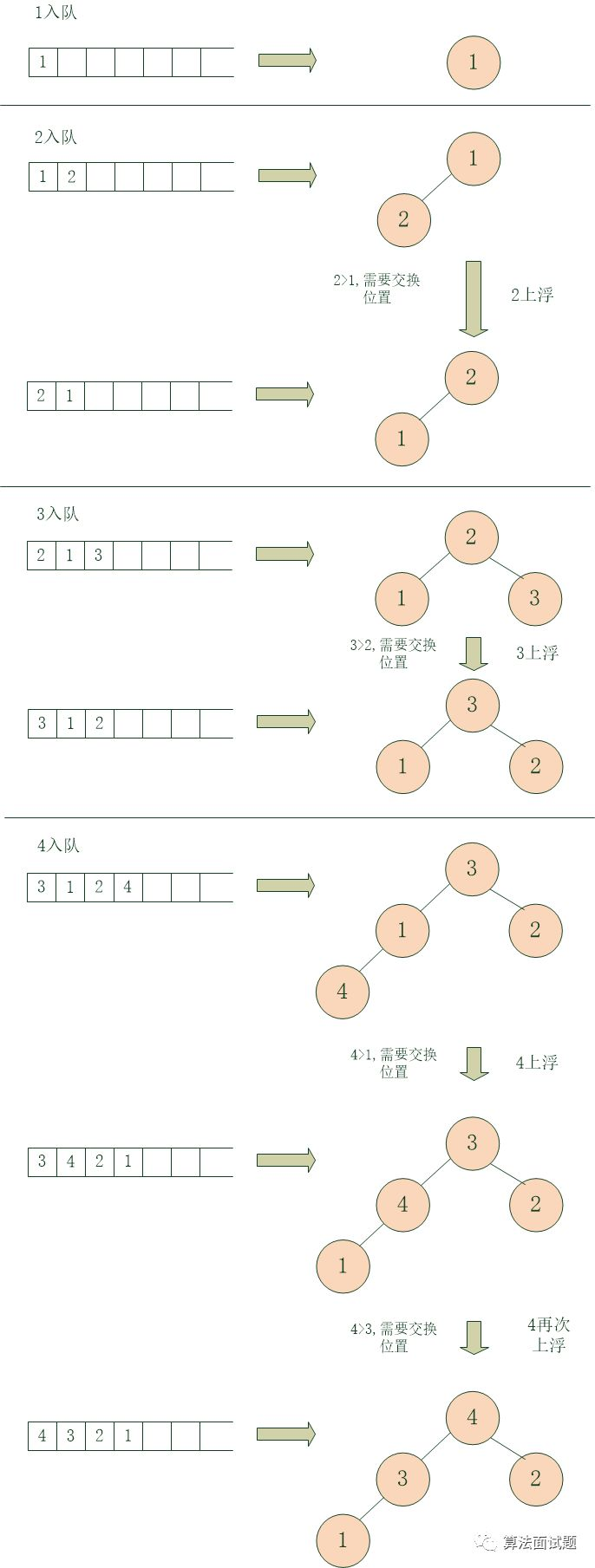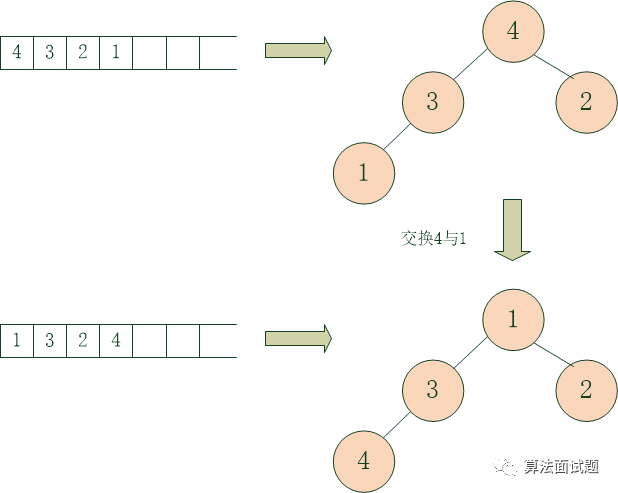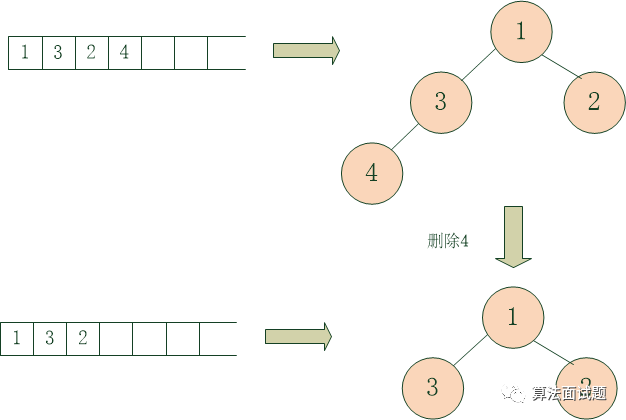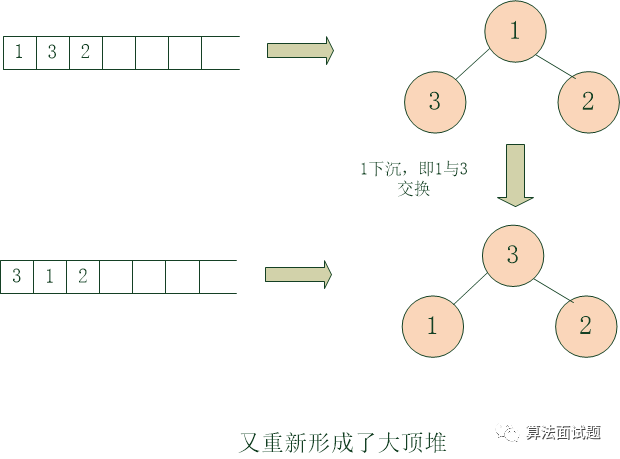# 问题

## 题目

dequeue（）出队操作，每调用一次从队列中找到一个优先级最高的元素出队，并返回此元素的值（val）

## 二叉堆的概念

### 入队### 出队# 源码实现

class PriorityQueue {
constructor() {
//数组，入队的元素保存在这里，
//每进行一次入队或出队操作，都需重新调整数组为大顶堆
this.arr = [];
}

//入队
enqueue(val, prior) {
this.arr.push({
val: val,
prior: prior
});
let cur = this.arr.length - 1;
let temp = this.arr[cur];
//对刚入队的那个元素实施上浮操作，即重新调整数组为大顶堆
for(let i = Math.floor((cur - 1) / 2); i >= 0; i = Math.floor((i - 1) / 2)) {
if(temp.prior > this.arr[i].prior) {
this.arr[cur] = this.arr[i];
cur = i;
} else break;
}
this.arr[cur] = temp;
}

//出队
dequeue() {
if(this.arr.length === 0) throw new Error("队列为空，不能出队");
//大顶堆保证了第一个元素的优先级永远最高，是要出队的元素

//将第一个元素的值缓存，以便返回
let res = this.arr.val;

//用队尾元素元素覆盖第一个元素
this.arr = this.arr[this.arr.length - 1];

//将队列长度-1
this.arr.length -= 1;

//重新调整队列为大顶堆
let cur = 0,
len = this.arr.length;
let temp = this.arr;
for(let i = 2 * cur + 1; i < len; i = 2 * cur + 1) {
if(i + 1 < len && this.arr[i].prior < this.arr[i + 1].prior)
i++;
if(temp.prior < this.arr[i].prior) {
this.arr[cur] = this.arr[i];
cur = i;
} else break;
}
this.arr[cur] = temp;

//返回结果
return res;
}
}

let p = new PriorityQueue();
p.enqueue(5, 6);
p.enqueue(2, 100);
p.enqueue(90, 1);

console.log(p.dequeue());//2
console.log(p.dequeue());//5
console.log(p.dequeue());//90